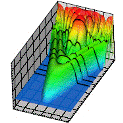## Research Papers in Physics and Astronomy## Anthony F. Starace Publications

April 1982

#### Comments

Published by American Physical Society. Phys. Rev. A 25, 2135 (1982). http://pra.aps.org. Copyright © 1982 American Physical Society. Permission to use.

#### Abstract

A graphical procedure is presented for evaluating each term in the equation satisfied by the first-order transition matrix for an arbitrary (open-shell) atom. For those terms involving the interaction of excited virtual pairs of electrons with the ionic core, we introduce the approximation that there is no exchange of orbital or spin angular momentum with the ionic core. This approximation is shown to lead to the random-phase approximation (RPA) equations in the closed-shell-atom case; we use it to define the RPA for open-shell atoms. The single equation satisfied by the first-order transition matrix is used to obtain a set of N+N′ coupled differential equations for N final-state radial functions and N initial-state radial functions, which together, completely determine the first-order transition matrix for an atomic system having N final-state channels. (The relation of N′ to N depends on the particular atom studied). The N+N′ differential equations are shown to reduce to familiar forms in the following cases: (1) When initial-state correlations are ignored, one obtains the close-coupling equations and (2) when closed-shell atoms are considered, N=N′ and one obtains the 2N coupled differential equations of the Chang-Fano version of the RPA. Finally, our RPA first-order transition matrix is used to evaluate the matrix element of the electric-dipole operator for an arbitrary (open-shell) atom. These RPA electric-dipole transition matrix elements may be used to calculate nonrelativistically all experimentally observable quantities resulting from a single-electron atomic photoabsorption process.

COinS